Courses

# Chapter 4 Gear Trains - Theory of Machine, Mechanical Engineering Mechanical Engineering Notes | EduRev

## Mechanical Engineering : Chapter 4 Gear Trains - Theory of Machine, Mechanical Engineering Mechanical Engineering Notes | EduRev

The document Chapter 4 Gear Trains - Theory of Machine, Mechanical Engineering Mechanical Engineering Notes | EduRev is a part of the Mechanical Engineering Course Mechanical Engineering SSC JE (Technical).
All you need of Mechanical Engineering at this link: Mechanical Engineering

GEAR TRAINS

A Gear Train is a combination of gears used to transmit motion from one shaft to another. It is required to obtain large speed reduction within a small space.

TYPE OF GEAR TRAIN
(i) Simple gear train: In this each shaft support one gear.
(ii) Compound gear train: In this each shaft support two gear wheels except first and last.
(iii) Reverted gear train: In this driving and the driven gear coaxial or coincident.
(iv) Planetary and epicyclic gear train

It is also possible that in a gear train, the axes of some of the wheels are not fixed but rotate around the axes of other wheels with which they mesh. Such trains are known as planetary or epicyclic gear trains. Epicyclic gear trains are useful to transmit very high velocity ratios with gear of smaller sizes in a lesser space.

SIMPLE GEAR TRAIN

• A series of gears, capable of receiving and transmitting motion from one gear to another is called a simple gear train. In it, all the gear axes remain fixed relative to the frame and each gear is on a separate shaft.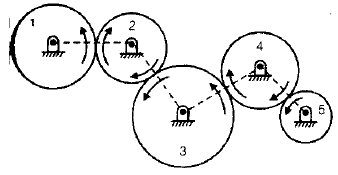• A pair of mated external gear always move in opposite direction.
• All odd numbered gears move in one direction and all even numbered gears in the opposite direction.
• A simple gear train can also have bevel gears.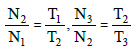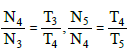Train value =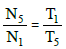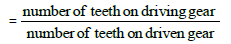Speed Ratio =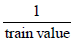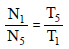•  Intermediate gears have no effect on the speed ratio and therefore, they are known as idlers.

COMPOUND GEAR TRAIN

• When a series of gears are connected in such a way that two or more gears rotate about an axis with the same angular velocity; it is known as compound gear train.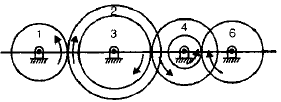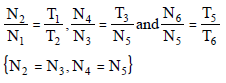Train Value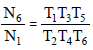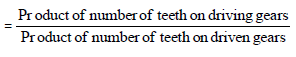Speed Ratio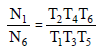REVERTED GEARTRAIN

•  If the axis of the first and last wheel of a compound gear coincide it is called reverted gear train.
•  Such arrangement is used in clock and in simple lathe where 'back gear' is used to give slow speed to the chuck.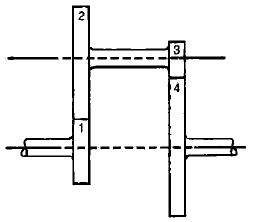•  Train value =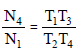If r is the pitch circle radius of a gear,
r1 + r2 = r3 + r4

EPICYCLIC GEAR TRAIN

• When there exists a relative motion of axes in a gear train, it is called an epicyclic gear train. Thus in an epicyclic train, the axis of at least one of the gears also move relative to the frame.
• Large speed reductions are possible with epicyclic gears and if the fixed wheel is annular a more compact unit could be obtained.
• Important applications of epicyclic gears are in transmission, computing devices.
• In general gear trains have two degrees of freedom.

DIFFERENTIAL GEAR

• When a vehicles takes a turn, the outer wheels must travel farther than the inner wheels. Since, Both rear wheels are driven by the engine through gearing. Therefore some sort of automatic device is necessary so that the two rear wheels are driven at slightly different speeds. This is accomplished by fitting differential gear on the rear axle.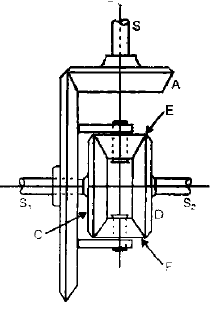• An epicyclic gear having two degrees of freedom has been utilized in the differential gear of an automobile. It permits the two wheels to rotate at the same speed when driving straight while allowing the wheels to rotate at different speeds when taking a turn. Thus, a differential gear is a device which adds or subtracts angular displacement.
Offer running on EduRev: Apply code STAYHOME200 to get INR 200 off on our premium plan EduRev Infinity!

83 docs|53 tests

,

,

,

,

,

,

,

,

,

,

,

,

,

,

,

,

,

,

,

,

,

,

,

,

;